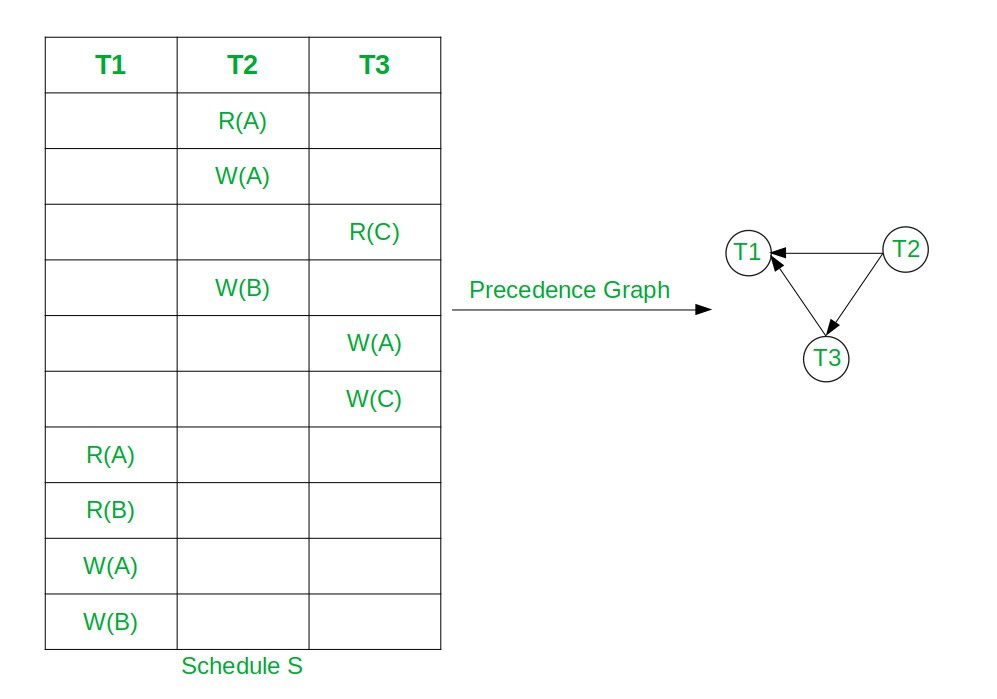# Equivalent Serial Schedule of Conflict Serializable Schedule in DBMS

• Difficulty Level : Hard
• Last Updated : 30 Sep, 2021

Prerequisite: Conflict Serializability, Precedence Graph

Conflict Serializable Schedule: A schedule is called conflict serializable if it can be transformed into a serial schedule by swapping non-conflicting operations. The serial schedule of the Conflict Serializable Schedule can be found by applying topological Sorting on the Precedence Graph of the Conflict Serializable Schedule.

Note: Precedence Graph of Conflict Serial Schedule is always directed acyclic graph.

Approach: Follow to below steps to find topological sorting of Precedence Graph:

• Find the indegree of all nodes for the given Precedence Graph and store it in an auxiliary array.
• Now For each node having indegree 0 perform the following:
• Print the current node T as the order of the topological sort.
• Let the node T be the node with in-degree 0.
• Remove T and all edges connecting to T from the graph.
• Update indegree of all nodes after the above steps.
• After the above steps, the topological sort of the given precedence graph can be calculated.

Below is the illustration of the above approach:

Let, the Conflict Serial Schedule be S: R2(A) W2(A) R3(C) W2(B) W3(A) W3(C) R1(A) R2(B) W1(A) W2(B)• Here node T2 has indegree 0.
• So, select T2 and remove T2 and all edges connecting from it.
• Now T3 has indegree 0. So, select T3 and remove the edge T3→T1.
• At the end select T3. So the topological Sorting is T2, T3, T1.
• Hence, the equivalent serial schedule of given conflict serializable schedule is T2→T3→T1, i.e., S2: R2(A) W2(A) W2(B) R3(C) W3(A) W3(C) R1(A) R2(B) W1(A) W1(B).

There may be more than one equivalent serial schedule of the conflict serializable schedule.

Below is the implementation to get the Serial Schedule of CSS using Topological Sorting:

## C++

 `// C++ program to print serial schedule` `// of CSS using Topological sorting` `#include ` `using` `namespace` `std;`   `class` `PrecedenceGraph {`   `    ``// No. of vertices` `    ``int` `V;`   `    ``// Pointer to an array containing` `    ``// adjacency vector` `    ``vector<``int``>* adj;`   `    ``// Vector to store SerialSchedule` `    ``vector<``int``> serialSchedule;`   `    ``// Function  declarations` `    ``void` `computeIndegree(``int``* indegree);` `    ``int` `findZeroIndegree(``int``* indegree,` `                         ``bool``* visited);`   `public``:` `    ``// Constructor` `    ``PrecedenceGraph(``int` `V);`   `    ``// Function declarations` `    ``void` `addEdge(``int` `v, ``int` `w);` `    ``void` `topologicalSort();` `    ``void` `printSchedule();` `};`   `// Function to create the precedence` `// graph` `PrecedenceGraph::PrecedenceGraph(``int` `V)` `{` `    ``this``->V = V;` `    ``adj = ``new` `vector<``int``>[V];` `}`   `// Function to add the edge to the` `// precedence graph` `void` `PrecedenceGraph::addEdge(``int` `v,` `                              ``int` `w)` `{` `    ``adj[v].push_back(w);` `}`   `// Function to compute indegree of nodes` `void` `PrecedenceGraph::computeIndegree(` `    ``int``* indegree)` `{` `    ``for` `(``int` `i = 1; i < V; i++) {`   `        ``// Traverse the adjacency list` `        ``// of node i` `        ``for` `(``int` `j = 0;` `             ``j < adj[i].size(); j++) {` `            ``int` `node = adj[i][j];`   `            ``// Update the indegree` `            ``// of node` `            ``indegree[node]++;` `        ``}` `    ``}` `}`   `// Function to find node with` `// zero indegree` `int` `PrecedenceGraph::findZeroIndegree(` `    ``int``* indegree, ``bool``* visited)` `{` `    ``for` `(``int` `i = 1; i < V; i++) {`   `        ``// If node is not visited` `        ``// and have indegree 0` `        ``if` `(!visited[i]` `            ``&& indegree[i] == 0) {`   `            ``// Mark node visited` `            ``visited[i] = ``true``;`   `            ``// Return node` `            ``return` `i;` `        ``}` `    ``}`   `    ``// All nodes are visited` `    ``return` `-1;` `}`   `// Function to find the topological` `// Sorting of the given graph` `void` `PrecedenceGraph::topologicalSort()` `{` `    ``// Create and initialize` `    ``// visited array` `    ``bool``* visited = ``new` `bool``[V]();`   `    ``// Create and initialize` `    ``// indegree array` `    ``int``* indegree = ``new` `int``[V]();`   `    ``computeIndegree(indegree);`   `    ``// Check if the node with` `    ``// indegree 0 is available` `    ``int` `node = findZeroIndegree(` `        ``indegree, visited);`   `    ``bool` `nodeAvailable = ``false``;`   `    ``if` `(node != -1) {` `        ``nodeAvailable = ``true``;` `    ``}` `    ``while` `(nodeAvailable) {`   `        ``// Add node to serial schedule` `        ``serialSchedule.push_back(node);`   `        ``for` `(``int` `i = 0;` `             ``i < adj[node].size(); i++) {`   `            ``// Delete all edges of current` `            ``// node and update indegree` `            ``indegree[adj[node][i]]--;` `        ``}`   `        ``// Find next node with indegree 0` `        ``node = findZeroIndegree(indegree,` `                                ``visited);`   `        ``if` `(node == -1) {`   `            ``// Node with zero indegree` `            ``// not available` `            ``nodeAvailable = ``false``;` `        ``}` `    ``}` `}`   `// Function to print the serial schedule` `void` `PrecedenceGraph::printSchedule()` `{` `    ``for` `(``int` `i : serialSchedule) {` `        ``cout << ``"T"` `<< i << ``" "``;` `    ``}` `}`   `// Driver Code` `int` `main()` `{` `    ``// Create a precedence graph` `    ``// given in the above diagram` `    ``PrecedenceGraph graph(4);` `    ``graph.addEdge(2, 1);` `    ``graph.addEdge(2, 3);` `    ``graph.addEdge(3, 1);`   `    ``// Find topological ordereing` `    ``graph.topologicalSort();`   `    ``// Print Schedule` `    ``cout << ``"Equivalent Serial"` `         ``<< ``" Schedule is :"``;` `    ``graph.printSchedule();` `}`

Output:

`Equivalent Serial Schedule is :T2 T3 T1`

Time Complexity: O(N)
Auxiliary Space: O(N)

My Personal Notes arrow_drop_up
Recommended Articles
Page :